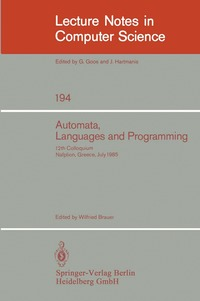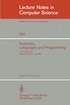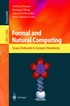Fler böcker inom
Format
Språk
Engelska
Antal sidor
524
Utgivningsdatum
1985-06-01
Upplaga
1985 ed.
Förlag
Springer-Verlag Berlin and Heidelberg GmbH & Co. K
Medarbetare
Brauer, Wilfried (ed.)
Illustrationer
XII, 524 p.
Dimensioner
234 x 156 x 27 mm
Vikt
745 g
Antal komponenter
1
Komponenter
1 Paperback / softback
ISBN
9783540156505

### Du kanske gillar# Automata, Languages and Programming

12th Colloquium, Nafplion, Greece, July 15-19, 1985. Proceedings

609
Skickas inom 10-15 vardagar.
Fri frakt inom Sverige för privatpersoner.
Vertex packing algorithms.- Linear and branching structures in the semantics and logics of reactive systems.- About rational sets of factors of a bi-infinite word.- A fair protocol for signing contracts.- The influence of key length on the area-time complexity of sorting.- Repeated synchronous snapshots and their implementation in CSP.- On total regulators generated by derivation relations.- Optimal solutions for a class of point retrieval problems.- Fractional cascading: A data structuring technique with geometric applications.- Hierarchies of one-way multihead automata languages.- Partitioning point sets in 4 dimensions.- A completeness theorem for recursively defined types.- Categorical combinatory logic.- Towards a uniform topological treatment of streams and functions on streams.- Infinite streams and finite observations in the semantics of uniform concurrency.- Imposed-functional dependencies inducing horizontal decompositions.- Characterization of high level tree transducers.- Ambiguity and transcendence.- A fast algorithm for polygon containment by translation.- Deterministic and Las Vegas primality testing algorithms.- Efficient algorithms for graphic matroid intersection and parity.- Operational semantics for order-sorted algebra.- A universal domain technique for profinite posets.- A simple proof of the Skolem-Mahler-Lech theorem.- On complete problems for NP?CoNP.- An algebraic theory of fair asynchronous communicating processes.- Hoare's logic for nondeterministic regular programs: A nonstandard completeness theorem.- Powerdomains as algebraic lattices preliminary report.- Random generation of combinatiorial structures from a uniform distribution.- Do rational equivalence relations have regular cross-sections?.- On probabilistic time and space.- The nearest neighbor problem on bounded domains.- Routing through a generalized switchbox.- On k-repetition free words generated by length uniform morphisms over a binary alphabet.- Every commutative quasirational language is regular.- A probabilistic distributed algorithm for set intersection and its analysis.- Distributed algorithms in synchronous broadcasting networks.- A context dependent equivalence between processes.- Lower bounds by kolmogorov-complexity.- A bidirectional shortest-path algorithm with good average-case behavior (preliminary version).- Applications of an infinite squarefree CO-CFL.- Special relations in automated deduction.- Dynamic interpolation search.- Polynomial levelability and maximal complexity cores.- Finite group topology and p-adic topology for free monoids.- On the use of relational expressions in the design of efficient algorithms.- The complementation problem for B chi automata with applications to temporal logic.- A complete compositional modal proof system for a subset of CCS.- On matrix multiplication using array processors.- Optimal parallel pattern matching in strings.- Compositionality and concurrent networks: Soundness and completeness of a proofsystem.

## Passar bra ihop

1.2. +
3.De som köpt den här boken har ofta också köpt Formal and Natural Computing av Wilfried Brauer, Hartmut Ehrig, Juhani Karhumaki, Arto K Salomaa (häftad).

## Kundrecensioner

Har du läst boken? Sätt ditt betyg »

## Innehållsförteckning

Vertex packing algorithms.- Linear and branching structures in the semantics and logics of reactive systems.- About rational sets of factors of a bi-infinite word.- A fair protocol for signing contracts.- The influence of key length on the area-time complexity of sorting.- Repeated synchronous snapshots and their implementation in CSP.- On total regulators generated by derivation relations.- Optimal solutions for a class of point retrieval problems.- Fractional cascading: A data structuring technique with geometric applications.- Hierarchies of one-way multihead automata languages.- Partitioning point sets in 4 dimensions.- A completeness theorem for recursively defined types.- Categorical combinatory logic.- Towards a uniform topological treatment of streams and functions on streams.- Infinite streams and finite observations in the semantics of uniform concurrency.- Imposed-functional dependencies inducing horizontal decompositions.- Characterization of high level tree transducers.- Ambiguity and transcendence.- A fast algorithm for polygon containment by translation.- Deterministic and Las Vegas primality testing algorithms.- Efficient algorithms for graphic matroid intersection and parity.- Operational semantics for order-sorted algebra.- A universal domain technique for profinite posets.- A simple proof of the Skolem-Mahler-Lech theorem.- On complete problems for NP?CoNP.- An algebraic theory of fair asynchronous communicating processes.- Hoare's logic for nondeterministic regular programs: A nonstandard completeness theorem.- Powerdomains as algebraic lattices preliminary report.- Random generation of combinatiorial structures from a uniform distribution.- Do rational equivalence relations have regular cross-sections?.- On probabilistic time and space.- The nearest neighbor problem on bounded domains.- Routing through a generalized switchbox.- On k-repetition free words generated by length uniform morphisms over a binary alphabet.- Every commutative quasirational language is regular.- A probabilistic distributed algorithm for set intersection and its analysis.- Distributed algorithms in synchronous broadcasting networks.- A context dependent equivalence between processes.- Lower bounds by kolmogorov-complexity.- A bidirectional shortest-path algorithm with good average-case behavior (preliminary version).- Applications of an infinite squarefree CO-CFL.- Special relations in automated deduction.- Dynamic interpolation search.- Polynomial levelability and maximal complexity cores.- Finite group topology and p-adic topology for free monoids.- On the use of relational expressions in the design of efficient algorithms.- The complementation problem for Buchi automata with applications to temporal logic.- A complete compositional modal proof system for a subset of CCS.- On matrix multiplication using array processors.- Optimal parallel pattern matching in strings.- Compositionality and concurrent networks: Soundness and completeness of a proofsystem.